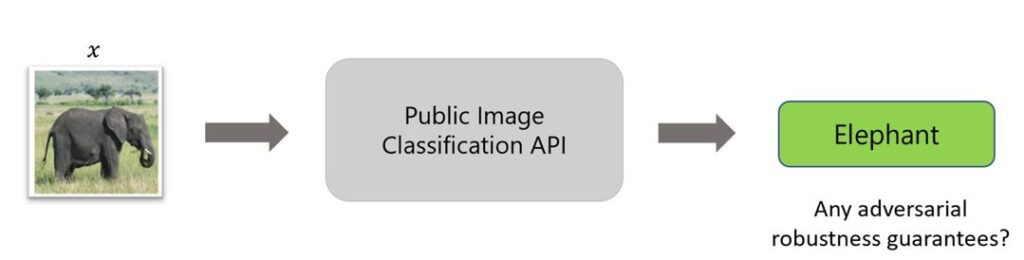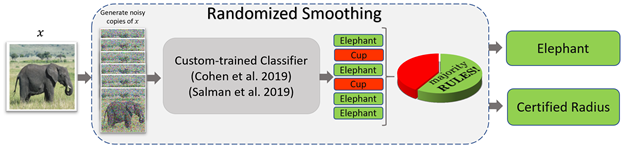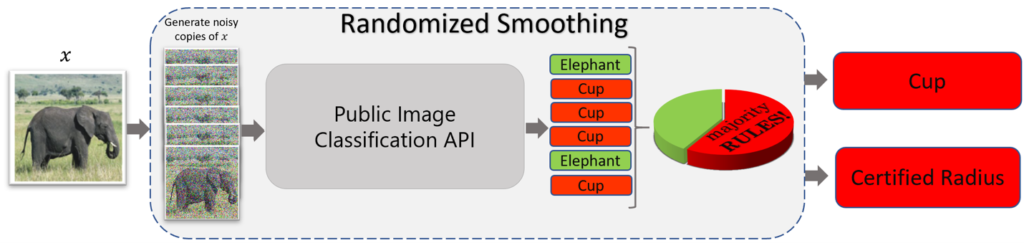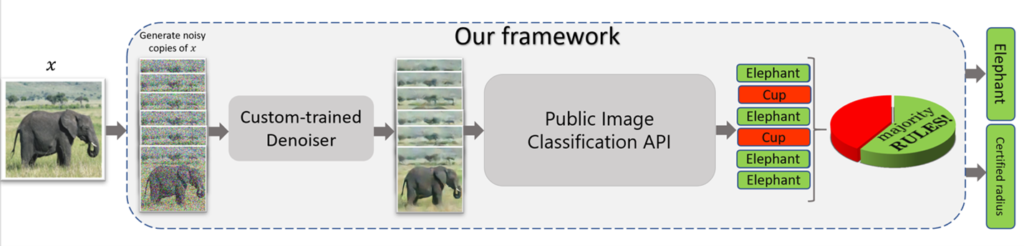# Denoised smoothing: Provably defending pretrained classifiers against adversarial examples

This post has been republished via RSS; it originally appeared at: Microsoft Research.

Editor’s note: This post and its research are the result of the collaborative efforts of a team of researchers comprising former Microsoft Research Engineer Hadi Salman, CMU PhD student Mingjie Sun, Researcher Greg Yang, Partner Research Manager Ashish Kapoor, and CMU Associate Professor J. Zico Kolter.

It’s been well-documented that subtle modifications to the inputs of image classification systems can lead to bad predictions. Take, for example, a model trained to classify images of an elephant. The model easily classifies an image of the animal grazing in a grassy field. Now, if just a few pixels in that image are maliciously altered, you can get a very different—and wrong—prediction despite the image appearing unchanged to the human eye. Sensitivity to such input perturbations, which are known as adversarial examples, raises security and reliability issues for the vision-based systems that we deploy in the real world. To tackle this challenge, recent research has revolved around building defenses against such adversarial examples. However, most of these adversarial defenses, such as randomized smoothing, require specifically training a classifier with a custom objective, which can be computationally expensive.

We consider a slightly different scenario: can we generate a provably robust classifier from off-the-shelf pretrained classifiers without retraining them specifically for robustness? In the paper “Denoised Smoothing: A Provable Defense for Pretrained Classifiers,” which we presented at the 34th Conference on Neural Information Processing Systems (NeurIPS 2020), we introduce denoised smoothing. Via the simple addition of a pretrained denoiser, we can apply randomized smoothing to make existing pretrained classifiers provably robust against adversarial examples without custom training. We envision our method being particularly helpful to those who don’t have the ability or access to train a classifier from scratch, such as users of public image classification APIs.Figure 1: Can we get any robustness guarantees when using pretrained classifiers, like those deployed in public image classification APIs, while having only query access to them?

## Randomized smoothing: An effective provable defense for classifiers

At the core of our approach is randomized smoothing, a promising and provable adversarial defense that has been shown to scale to large networks and datasets. Essentially, randomized smoothing smooths out a classifier: it takes a “brittle,” or “jittery,” function and makes it more stable, helping to ensure predictions for inputs in the neighborhood of a specific data point are constant.

Consider, as described in our paper, “a classifier $$f$$ mapping inputs in $$R^d$$ to classes in $$Y$$; the randomized smoothing procedure converts the base classifier $$f$$ into a new, smoothed classifier $$g$$. Specifically, for input $$x$$, $$g$$ returns the class that is most likely to be returned by the base classifier $$f$$ under isotropic Gaussian noise perturbations” (which are required for randomized smoothing) of $$x$$:

\begin{align} g(x) = \arg\max_{c \in \mathcal{Y}} \; \mathbb{P}[f(x+\delta) = c] \label{eq:smoothed-hard} \quad \text{where} \; \delta \sim \mathcal{N}(0, \sigma^2 I) \; \end{align}

In the above, “the noise level $$\sigma$$ controls the tradeoff between robustness and accuracy: as $$\sigma$$ increases, the robustness of the smoothed classifier increases while its standard accuracy decreases.” In other words, as the classifier becomes more robust to perturbations, there could be a loss of accuracy on images that have no perturbations, requiring users to balance the tradeoff depending on the use case.

A team of researchers from Carnegie Mellon University (CMU) and the Bosch Center for Artificial Intelligence showed that the above procedure leads to a robustness guarantee against $$\ell_2$$ adversarial attacks, and subsequent works derived similar guarantees for other threat models.

## Randomized smoothing in practice

In practice, $$g(x)$$ is hard to calculate, so it’s estimated by querying the classifier multiple times with modified versions of an image. So given a data point $$x$$ for which we want a prediction using randomized smoothing, we would take the following steps:

1. Replicate n copies of the image, adding random Gaussian noise to each of them.

2. Query the base classifier $$f$$ at each of these data points to get a prediction $$y_i$$.

3. Take the majority vote of the $$y_i$$‘s as the final prediction of the smoothed classifier $$g$$ at $$x$$.Figure 2: To obtain a prediction using randomized smoothing, multiple noisy copies of the image of interest are generated. A classifier trained to classify well under noisy inputs is queried with the noisy copies, producing a prediction for each. The majority vote is then taken as the final prediction and accompanied by a robustness guarantee in the form of a certified radius.

For a base classifier $$f$$, one can apply the above procedure to get a prediction of any data point $$x$$ along with a robustness guarantee in the form of a certified radius, the radius around a given input for which the prediction is guaranteed to be fixed. So, for example, if you pass an image of an elephant with a specific certified radius into a classifier and then pass the same image with a small perturbation through the classifier and the new image falls within that radius, the classifier will give the same prediction for both.

But the above procedure assumes that the base classifier $$f$$ classifies well under Gaussian perturbations of its inputs. Specifically, in Step 3, we take the majority votes over noisy perturbation of the input $$x$$. So the base classifier $$f$$ must be trained in a way that classifies well under noisy inputs. Several papers have proposed techniques to train the base classifier $$f$$, including the CMU-Bosch Center for AI paper, which uses Gaussian noise data augmentation, and our 2019 NeurIPS paper, which uses adversarial training.

## Applying randomized smoothing to pretrained classifiers

Now what if the base classifier $$f$$ is some off-the-shelf classifier that wasn’t trained specifically for randomized smoothing—that is, it doesn’t classify well under noisy perturbations of its inputs? For example, what if we applied randomized smoothing to a public vision API? Do we get good robustness guarantees? Figure 3 illustrates what happens in this case. Basically, we get poor predictions and poor robustness guarantees since these APIs aren’t trained to classify well under substantial levels of noise added to their inputs (which, to reiterate, is required for randomized smoothing). Therefore, randomized smoothing leads to trivial guarantees when applied as is to these pretrained classifiers, as we demonstrate in our paper, and thus isn’t effective.Figure 3: If we apply randomized smoothing to off-the-shelf classifiers, bad results are obtained, as these classifiers don’t classify well when substantial noise levels are added to their inputs, which is required for randomized smoothing.

With our work on denoised smoothing, we make randomized smoothing effective for classifiers that aren’t trained specifically for randomized smoothing. Our proposed method is straightforward; as mentioned above, instead of applying randomized smoothing to these classifiers, we prepend a custom-trained denoiser in front of these classifiers and then apply randomized smoothing (Figure 4). The denoiser helps by removing noise from the synthetic noisy copies $$x$$+$$\delta_i$$ of the input $$x$$, which allows the pretrained classifiers to give better predictions, as they now see denoised images they’re able to classify better.Figure 4: Denoised smoothing, which adds a custom-trained denoiser before the classifier to be defended, makes randomized smoothing effective for classifiers that aren’t trained specifically for it. The denoiser helps by removing noise from the copies of the input, allowing a pretrained classifier to give better predictions, as it now sees denoised images it’s able to classify better.

## How to train the denoiser

In our paper, we investigate several ways for training the denoiser $$\mathcal{D}_{\theta}$$.

Mean square error objective: simply train the denoiser to minimize the mean square error (MSE) between the clean image and the denoised image

$$\begin{equation}L_{\text{MSE}}= \mathbb{E}_{\mathcal{S}, \delta}||\mathcal{D}_{\theta}(x_i + \delta)-x_i||_{2}^{2}\end{equation}$$

Stability objective: given a classifier $$F$$ attached to the denoiser $$\mathcal{D}_{\theta}$$, minimize the cross-entropy loss between the prediction of $$F(\mathcal{D}_{theta})$$ at the noisy input $$x_i + \delta$$ and the prediction of the classifier at the clean data point $$x_i$$

\begin{align}L_{\text{Stab}}&=\mathbb{E}_{\mathcal{S}, \delta} \mathcal{\ell_\text{CE}}(F(\mathcal{D}_\theta(x_i + \delta)), f(x_i)) \quad \text{where} \; \delta \sim \mathcal{N}(0, \sigma^2 I) \; \end{align}

MSE-stability hybrid: train the denoiser with MSE, then fine-tune it with the stability objective

## Improvements in certified accuracy

Our method allows us to boost the certified accuracy of a ResNet-110 pretrained on CIFAR-10 and ResNet-18/34/50 classifiers pretrained on ImageNet as shown in the tables below. We test our method in two scenarios. In the white-box scenario, the pretrained classifier is assumed to be known, which allows for the exploration of more ways to train the denoiser. In theory, since we know the specific classifier being used, we can backpropagate gradients through it to update our denoiser. In the black-box scenario, the pretrained classifier is not known; we don’t have any information about its architecture or parameters. We compare our method deployed in these scenarios to a “no denoiser” baseline, which applies randomized smoothing to these pretrained classifiers directly without any denoising step. We observe substantial improvements in the robustness guarantee (certified accuracy) at various $$\ell_2$$ radii (the higher the certified accuracy, the more robust the classifier is).

Finally, we apply denoised smoothing to four public vision APIs and show how we can get certified predictions using these APIs without having access to the underlying models at all (see the paper for the results). While our method can help make possible the protection of vision-based systems from adversarial examples without having to custom train the pretrained classifiers powering them, this work is only a starting point in making pretrained classifiers provably robust. Next steps for denoised smoothing can include working to achieve even stronger guarantees, particularly in scenarios in which the classifier being used is not known.

This site uses Akismet to reduce spam. Learn how your comment data is processed.